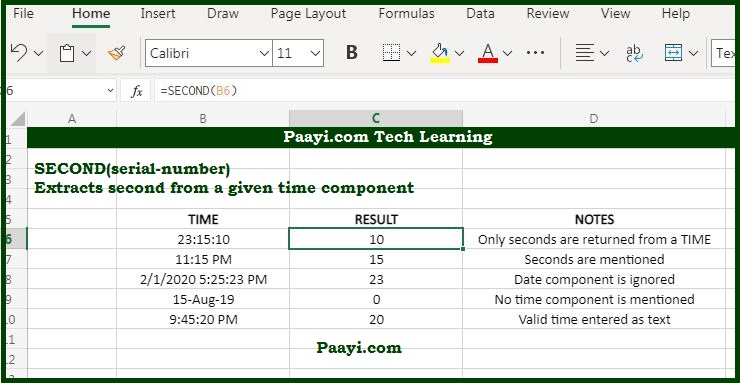# Learn How to Use Microsoft Excel SECOND Function

Written by | 0 Comments | 558 Views

In this article, you will learn how to use the Microsoft Excel SECOND function and its prime function in Microsoft Excel. You will also get to know the Microsoft Excel SECOND function return value and syntax with the help of some examples.

## Microsoft Excel SECOND Function

The Microsoft Excel SECOND function is used to get a second number (0-59) from a time. That means the SECOND function returns the second component of the specified time with return value as a number between 0 to 59.

Let us understand this by the example, with a given time 10:15:20 AM; the SECOND function will return the value 20. The SECOND function can be used to extract a second into a cell and can also be used to feed the result into another formula, like the TIME function.

### SECOND Function Return Value

The return value will be the number 0 to 59.

### SECOND Function Syntax

= SECOND(serial-number)

Where:

• A valid Excel time format.

## How to Use Microsoft Excel SECOND Function?You can supply the time as a text, like - "8:45 PM" or as a decimal number as 0.5, which implies 12:00 PM. Excel stores dates and time as a serial number as the date Jan 1, 2000, 12:00 PM is the same as the serial number - 32526.5 in Excel. If you want to check whether Excel correctly recognizes the date or time, you format the date as a number temporarily.